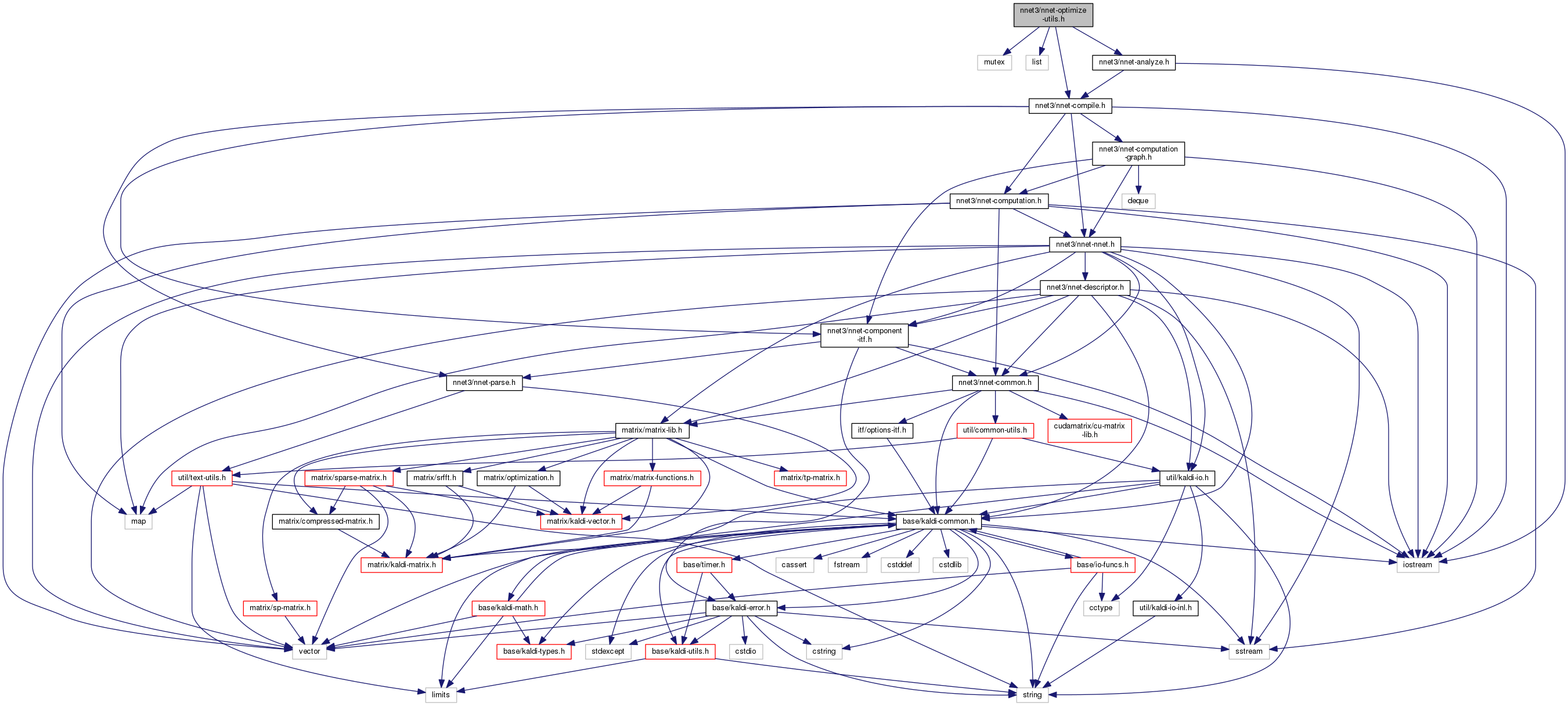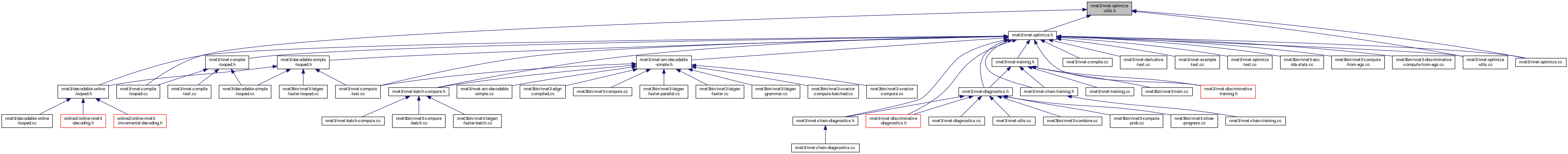nnet-optimize-utils.h File Reference
`#include <mutex>`
`#include <list>`
`#include "nnet3/nnet-compile.h"`
`#include "nnet3/nnet-analyze.h"`
Include dependency graph for nnet-optimize-utils.h:This graph shows which files directly or indirectly include this file:Go to the source code of this file.

## Classes

class  VariableMergingOptimizer
This class is responsible for merging matrices, although you probably want to access it via the the function VariableMergingOptimization(). More...

class  DerivativeTimeLimiter

struct  DerivativeTimeLimiter::MatrixPruneInfo

class  ComputationCache
Class ComputationCache is used inside class CachingOptimizingCompiler to cache previously computed computations. More...

## Namespaces

kaldi
This code computes Goodness of Pronunciation (GOP) and extracts phone-level pronunciation feature for mispronunciations detection tasks, the reference:

kaldi::nnet3

## Functions

void ExtendMatrices (NnetComputation *computation)
This is not really an optimization in itself but it can make things easier for class VariableMergingOptimizer (usually called by its wrapper VariableMergingOptimization()). More...

void ConsolidateModelUpdate (const Nnet &nnet, NnetComputation *computation)
This optimization consolidates the model-update part of backprop commands, for components in (e.g.) recurrent networks that need to have many separate backprop commands, into more efficient single commands operating on consolidated data in larger matrices. More...

int32 MaxOutputTimeInRequest (const ComputationRequest &request)

void LimitDerivativeTimes (const Nnet &nnet, int32 min_deriv_time, int32 max_deriv_time, NnetComputation *computation)

bool RequestIsDecomposable (const ComputationRequest &request, ComputationRequest *mini_request, int32 *num_n_values)
This function, used in 'shortcut' compilation where we first compile a smaller computation with the same structure but only 2 distinct 'n' values, works out whether a computation is 'decomposable'; if so, it returns true and outputs the 'mini_request' with the same structure, and the number of 'n' values. More...

void ExpandComputation (const Nnet &nnet, const MiscComputationInfo &misc_info, const NnetComputation &computation, bool need_debug_info, int32 num_n_values, NnetComputation *expanded_computation)
This function is used in 'shortcut' compilation to expand a computation that has been compiled for exactly 2 'n' values, to one that is suitable for some num_n_values > 2. More...

bool ReplaceRowWithMatrixOps (NnetComputation *computation)
This function detects cases where commands of type kCopyRows, kAddRows or kAddToRows can be converted to commands of type kMatrixCopy or kMatrixAdd, and converts them (this may involve adding submatrices). More...

bool SnipRowOps (NnetComputation *computation)
This function detects cases where commands of type kCopyRows, kAddRows, kAddRowsMulti, kAddToRowsMulti, kCopyRowsMulti, kCopyToRowsMulti or kAddRowRanges use indexes that start or end with -1's or equivalents, and replace them with similar commands that act on a sub-matrix of the matrices they are currently acting on. More...

bool SplitRowOps (NnetComputation *computation)
This function detects cases where commands of type kAddRowsMulti, kAddToRowsMulti, kCopyRowsMulti, kCopyToRowsMulti use indexes that correspond to at most two submatrices, in two distinct ranges without gaps filled by -1's, and could be converted to at most two commands of type kMatrixAdd, kMatrixCopy, kAddRows or kCopyRows. More...

void RenumberComputation (NnetComputation *computation)
This function detects submatrices and matrices that are never used (e.g. More...

void RemoveNoOps (NnetComputation *computation)
Removes commands of type kNoOperation in the computation. More...

void IdentifySubmatrixArgs (NnetComputation::Command *command, std::vector< int32 * > *submatrix_args)
This function outputs to "submatrix_args" the addresses of a subset of arguments arg1 through arg6 in "command", that correspond to the indexes of submatrices. More...

bool MatrixIsUnused (const Analyzer &analyzer, const NnetComputation &computation, int32 m)
This function returns true if matrix 1 <= m < computation->matrices.size() is unused, defined as: it is not an input or an output, and is not accessed other than via commands of type kAllocMatrix, kDeallocMatrix, and kSetConst. More...

void RemoveCommandsForUnusedMatrix (const Analyzer &analyzer, int32 m, NnetComputation *computation)
This function removes from 'computation' the commands accessing matrix 'm', which is assumed to be unused according to the MatrixIsUnused() command above. More...

void IdentifySubmatrixArgs (std::vector< NnetComputation::Command > *commands, std::vector< int32 * > *submatrix_args)
This function outputs to "submatrix_args" the addresses of the args (arguments arg1 through arg6) in the vector "commands", that correspond to the indexes of submatrices. More...

void IdentifySubmatrixArgsInComputation (NnetComputation *computation, std::vector< int32 * > *submatrix_args)
This function outputs to "submatrix_args" the addresses of integers in 'computation' that correspond to submatrices. More...

void IdentifyIndexesMultiArgs (std::vector< NnetComputation::Command > *commands, std::vector< int32 * > *indexes_multi_args)
Identifies in the vector of commands, arguments that correspond to indexes into the computation's indexes_multi array, and outputs a list of pointers to those arguments to 'indexes_multi_args'. More...

void IdentifyIndexesArgs (std::vector< NnetComputation::Command > *commands, std::vector< int32 * > *indexes_args)
Identifies in the vector of commands, arguments that correspond to indexes into the computation's 'indexes' array, and outputs a list of pointers to those arguments to 'indexes_args'. More...

void IdentifyIndexesRangesArgs (std::vector< NnetComputation::Command > *commands, std::vector< int32 * > *indexes_ranges_args)
Identifies in the vector of commands, arguments that correspond to indexes into the computation's 'indexes_ranges' array, and outputs a list of pointers to those arguments to 'indexes_ranges_args'. More...

void InsertCommands (std::vector< std::pair< int32, NnetComputation::Command > > *commands, NnetComputation *computation)
Inserts commands into the computation at the requested places. More...

void OptimizeMemoryCompression (const Nnet &nnet, int32 memory_compression_level, NnetComputation *computation)
Performs optimization to reduce memory usage where possible, making use of the kCompressMatrix and kDecompressMatrix commands. More...

void OptimizeLoopedComputation (const Nnet &nnet, NnetComputation *computation)
This function tries to optimize computation 'computation' for an 'looped' computation. More...

void FixGotoLabel (NnetComputation *computation)
This function ensures that the arg1 of a final command of type kGotoLabel is the same as the command with type kNoOperationLabel. More...